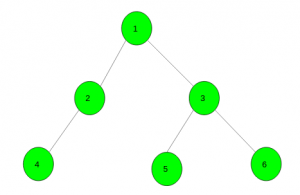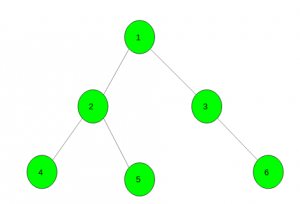Minimum steps to color the tree with given colors

• Difficulty Level : Hard
• Last Updated : 03 Jun, 2021

Given a tree with N nodes which initially have no color and an array color[] of size N which represent the color of each node after the coloring process takes place. The task is to color the tree into the given colors using the smallest possible number of steps. On each step, one can choose a vertex v and a color x, and then color all vertices in the sub-tree of v (including v itself) with color x. Note that root is vertex number 1.
Examples:

Input: color[] = { 1, 1, 2, 1, 3, 1}Output:
Color the sub-tree rooted at node 1 with color 1.
Then all the vertices have colors 1.
Now, color the sub-tree rooted at 3 with color 2.
Finally, color the sub-trees rooted at 5 and 6 with colors 3 and 1 respectively.
Input: color[] = { 1, 2, 3, 2, 2, 3}Output:

Approach: Call a DFS function at vertex 1 and initially keep answer as zero. Increment the answer whenever there is a difference in colors of child and parent nodes.
See the below code for better understanding.
Below is the implementation of the above approach:

C++

 // C++ implementation of the approach#include using namespace std; // To store the required answerint ans = 0; // To store the graphvector gr; // Function to add edgesvoid Add_Edge(int u, int v){    gr[u].push_back(v);    gr[v].push_back(u);} // Dfs functionvoid dfs(int child, int par, int color[]){     // When there is difference in colors    if (color[child] != color[par])        ans++;     // For all it's child nodes    for (auto it : gr[child]) {        if (it == par)            continue;        dfs(it, child, color);    }} // Driver codeint main(){     // Here zero is for parent of node 1    int color[] = { 0, 1, 2, 3, 2, 2, 3 };     // Adding edges in the graph    Add_Edge(1, 2);    Add_Edge(1, 3);    Add_Edge(2, 4);    Add_Edge(2, 5);    Add_Edge(3, 6);     // Dfs call    dfs(1, 0, color);     // Required answer    cout << ans;     return 0;}

Python3

 # Python3 implementation of the approach # To store the required answerans = 0 # To store the graphgr = [[] for i in range(100005)] # Function to add edgesdef Add_Edge(u, v):    gr[u].append(v)    gr[v].append(u) # Dfs functiondef dfs(child, par, color):    global ans     # When there is difference in colors    if (color[child] != color[par]):        ans += 1     # For all it's child nodes    for it in gr[child]:        if (it == par):            continue        dfs(it, child, color)     # Driver code # Here zero is for parent of node 1color = [0, 1, 2, 3, 2, 2, 3] # Adding edges in the graphAdd_Edge(1, 2)Add_Edge(1, 3)Add_Edge(2, 4)Add_Edge(2, 5)Add_Edge(3, 6) # Dfs calldfs(1, 0, color) # Required answerprint(ans) # This code is contributed# by mohit kumar